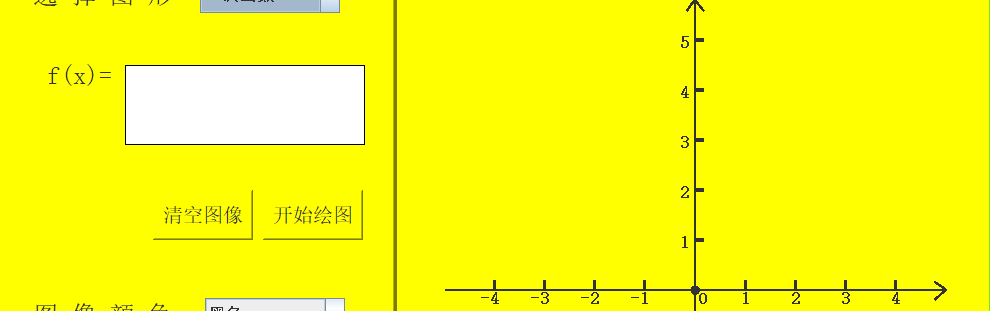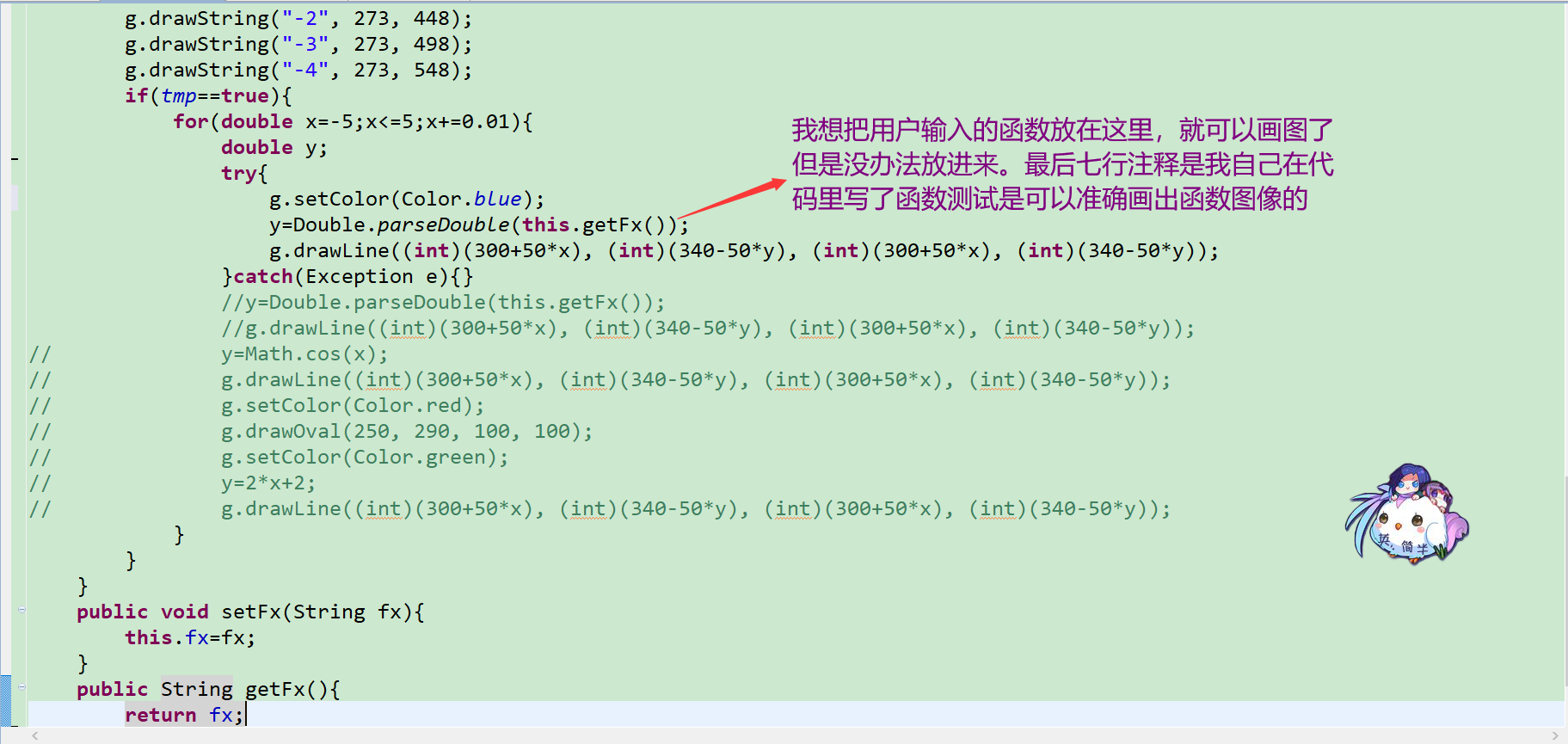# 大神们来帮帮忙...全文
99 点赞 收藏 8

8 条回复

for exsample
interface Fn { //定义函数接口
int fn(int... args);//函数方法
}
Fn fn; //定义函数接口变量
//String s = jtextarea.getText();
String s = “3x + 2”; //假设画面输入
String reg0 = “\\s*?([+-])\\s*?(\\d*?)\\s*”;
String reg1 = “^(\\d*?)([xX)(” + reg0+ “)\$”;
if (s.matches(reg1)) {
int a = Integer.parseInt(s.replacAll(reg1, “\$1”)); //正则提取3x + 2中的3
String s2 = s.replaceAll(reg1, “\$3”);
String ss = s2.replaceAll(reg0, ”\$1”); //正则提取3x + 2中的+
int b = Integer.parseInt(s2.replaceAll(reg0, “\$2”)); //提取3x +2中的2
if (“-“.equase(ss)) b = -b; //如果提取的+是-
fn = (a1, x, b1)->{ //函数接口实例化
return a1*x+b1;
};
for (int x=-5; x<=5; x++) { //x循环
int y = fn.fn(a, x, b); //计算y点
...//画线
}
}

qybao 2019年11月22日

qybao 2019年11月22日

qybao 2019年11月22日

for exsample
interface Fn { //定义函数接口
int fn(int... args);//函数方法
}
Fn fn; //定义函数接口变量
//String s = jtextarea.getText();
String s = “3x + 2”; //假设画面输入
String reg0 = “\\s*?([+-])\\s*?(\\d*?)\\s*”;
String reg1 = “^(\\d*?)([xX)(” + reg0+ “)\$”;
if (s.matches(reg1)) {
int a = Integer.parseInt(s.replacAll(reg1, “\$1”)); //正则提取3x + 2中的3
String s2 = s.replaceAll(reg1, “\$3”);
String ss = s2.replaceAll(reg0, ”\$1”); //正则提取3x + 2中的+
int b = Integer.parseInt(s2.replaceAll(reg0, “\$2”)); //提取3x +2中的2
if (“-“.equase(ss)) b = -b; //如果提取的+是-
fn = (a1, x, b1)->{ //函数接口实例化
return a1*x+b1;
};
for (int x=-5; x<=5; x++) { //x循环
int y = fn.fn(a, x, b); //计算y点
...//画线
}
}

weixin_40599844 2019年11月22日

for exsample
interface Fn { //定义函数接口
int fn(int... args);//函数方法
}
Fn fn; //定义函数接口变量
//String s = jtextarea.getText();
String s = “3x + 2”; //假设画面输入
String reg0 = “\\s*?([+-])\\s*?(\\d*?)\\s*”;
String reg1 = “^(\\d*?)([xX)(” + reg0+ “)\$”;
if (s.matches(reg1)) {
int a = Integer.parseInt(s.replacAll(reg1, “\$1”)); //正则提取3x + 2中的3
String s2 = s.replaceAll(reg1, “\$3”);
String ss = s2.replaceAll(reg0, ”\$1”); //正则提取3x + 2中的+
int b = Integer.parseInt(s2.replaceAll(reg0, “\$2”)); //提取3x +2中的2
if (“-“.equase(ss)) b = -b; //如果提取的+是-
fn = (a1, x, b1)->{ //函数接口实例化
return a1*x+b1;
};
for (int x=-5; x<=5; x++) { //x循环
int y = fn.fn(a, x, b); //计算y点
...//画线
}
}

3.4w+

30.7w+

Java 2 Standard Edition